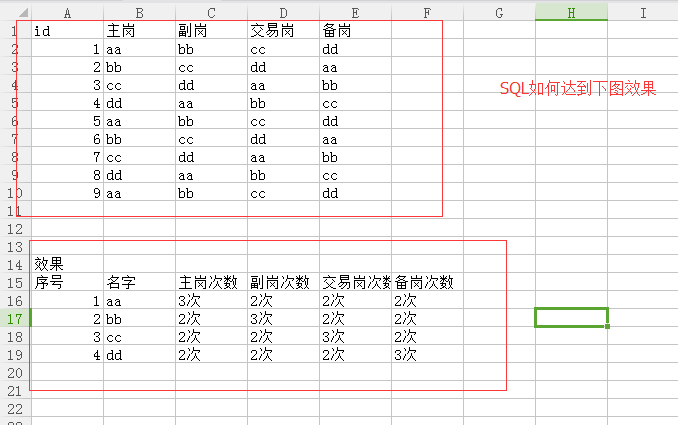MYSQL查询。。请教大神~~~2个回答

`````` select a1
,(select count(*) from 表 where 主岗=t.a1) 主岗次数
,(select count(*) from 表 where 副岗=t.a1) 副岗次数
,(select count(*) from 表 where 交易岗=t.a1) 交易岗次数
,(select count(*) from 表 where 备岗=t.a1) 备岗次数
from(
select distinct 主岗 a1 from 表
) t
``````